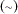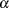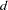The NLMIXED Procedure
 RANDOM Statement

RANDOM random-effectsdistribution SUBJECT=variable <options> ;

The RANDOM statement defines the random effects and their distribution. The random effects must be represented by symbols that appear in your SAS programming statements. They typically influence the mean value of the distribution specified in the MODEL statement. The RANDOM statement consists of a list of the random effects (usually just one or two symbols), a tilde, the distribution for the random effects, and then a SUBJECT= variable.

NOTE: The input data set must be clustered according to the SUBJECT= variable. One easy way to accomplish this is to sort your data by the SUBJECT= variable prior to calling PROC NLMIXED. PROC NLMIXED does not sort the input data set for you; rather, it processes the data sequentially and considers an observation to be from a new subject whenever the value of its SUBJECT= variable changes from the previous observation.

The only distribution available for the random effects is normal(,) with meanand variance.

This syntax is illustrated as follows for one effect:

```   random u ~ normal(0,s2u) subject=clinic;
```

For multiple effects, you should specify bracketed vectors forand, the latter consisting of the lower triangle of the random-effects variance matrix listed in row order. This is illustrated for two and three random effects as follows:

```   random b1 b2 ~ normal([0,0],[g11,g21,g22]) subject=person;
random b1 b2 b3 ~ normal([0,0,0],[g11,g21,g22,g31,g32,g33])
subject=person;
```

The SUBJECT= variable determines when new realizations of the random effects are assumed to occur. PROC NLMIXED assumes that a new realization occurs whenever the value of the SUBJECT= variable changes from the previous observation, so your input data set should be clustered according to this variable. One easy way to accomplish this is to run PROC SORT prior to calling PROC NLMIXED by using the SUBJECT= variable as the BY variable.

Only one RANDOM statement is permitted, so multilevel nonlinear mixed models are not accommodated. However, you can specify certain nested random effects structure with a single RANDOM statement (see Chapter 15 of Littell et al. (2006) for an example).

The following options are available in the RANDOM statement:

ALPHA=specifies the alpha level to be used in computingstatistics and intervals. The default value corresponds to the ALPHA= option in the PROC NLMIXED statement.

DF=specifies the degrees of freedom to be used in computingstatistics and intervals in the OUT= data set. The default value corresponds to the DF= option in the PROC NLMIXED statement.

OUT=SAS-data-set

requests an output data set containing empirical Bayes estimates of the random effects and their approximate standard errors of prediction.Previous Page | Next Page | Top of Page